• matlab标定程序使用的测试图片 棋盘状图片 tif格式
• 计算机视觉的基本任务之一是从摄像机获取的图像信息出发计算三维空间中物体的几何信息，并由此重建和识别物体。这两者之间的关系就是由摄像机的几何成像模型来决定，...这里提供了张正友和Tasi的两种标定方法的源程序
• matlab做的标定界面，包括角点检测与张正友标定算法做的小软件
• 加州理工大学matlab相机标定工具箱，程序注解。注解详细介绍了工具箱的变成原理，对于初学者理解工具箱十分有用
• 自编matlab程序+matlab标定助手程序+标定板图像 两种方法进行结果对比 自编程序可以直接运行
• 立体视觉的标定程序 matlab 希望对大家有所帮助
• MATLAB摄像机标定程序，对于做三维摄像机标定的人很有用，希望大家分享！
• Douskos V.等牛人基于通用的Bonguet 相机标定工具箱改进编写的matlab相机标定程序，不用像原来的工具箱那样需要手动提取角点，这个软件有现成的界面，且开源，20幅左右的图片标定只需要12s。
• 基于matlab的tsai标定程序，精度较高，注释详细。
• matlab投影仪标定matlab中的投影仪标定方法属于投射标定图案法，投射的图案只能是棋盘格，该方法将投影仪当作逆向的相机，它具有映射图像二维点到三维坐标的功能，因此可以使用张正友标定方法来对它进行标定。...
matlab投影仪标定matlab中的投影仪标定方法属于投射标定图案法，投射的图案只能是棋盘格，该方法将投影仪当作逆向的相机，它具有映射图像二维点到三维坐标的功能，因此可以使用张正友标定方法来对它进行标定。投影仪标定图像的获取获取图片的过程按照投射标定图案法博客中来做就可，需要注意的是，我推荐大家在进行投影仪标定之前先标定好相机，不要将相机和投影仪放在一起标定。因为，在投影仪标定中，需要考虑到投影仪投射区域是否在相机视场范围内来摆放标定板，因此标定板位姿的摆放受到了限制，可能会影响相机的标定精度。下图为拍摄投影仪标定图片的示例，对于手边资源不足的同学，只需要准备一张较为平整的白板(尺寸大一点，给投影仪投射图案留好区域)，将打印的标定板贴上去即可。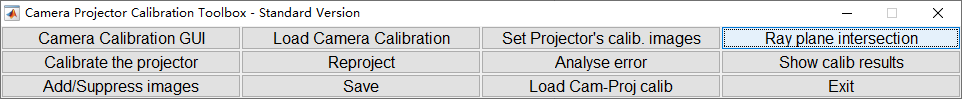在张正友的相机标定方法中，关键的输入参数有两个，第一个是角点的图像的坐标(在图像坐标系下)，第二个是标定板上角点的空间坐标(在世界坐标系下)。如果我们想要将张正友标定方法应用于投影仪标定，那么这两个关键参数如何获得呢？ 角点坐标的获取在使用投射标定图案法进行投影仪标定的系统中，输入参数中的角点图像坐标对于每组标定位姿来说都是一样的(就类似于相机标定法中，每组位姿的角点世界坐标都是一样的)，只需要对投射图案进行角点提取，获取的角点坐标就是该参数的值；角点空间坐标的获取首先，非常明确的是，单相机是无法恢复图像中任意像素点对应的深度信息的。因此，为了获取投影仪投射的棋盘格角点在相机坐标系下的三维坐标，需要创造回去深度信息的条件。那如何创建呢？毫无疑问，肯定是标定板了。因为，对于已标定的相机来说，我们可以计算出标定板平面上任意一点的坐标。因此，我们只需要将投射图案投射到标定板所在的平面就可获取角点的空间坐标。matlab投影仪标定方法在刚刚安装好的matlab中，是没有投影仪标定的的功能的，如果想要使用的话必须要要添加该工具箱(工具箱下载链接见星球)。在下载好该工具箱后，进行解压缩，并将该工具箱路径添加到matlab的搜索路径即可使用该功能。使用注意点1)在matlab命令行中，输入“cam_proj_gui”，即可将投影仪标定模块的ui界面调出来，如下图所示；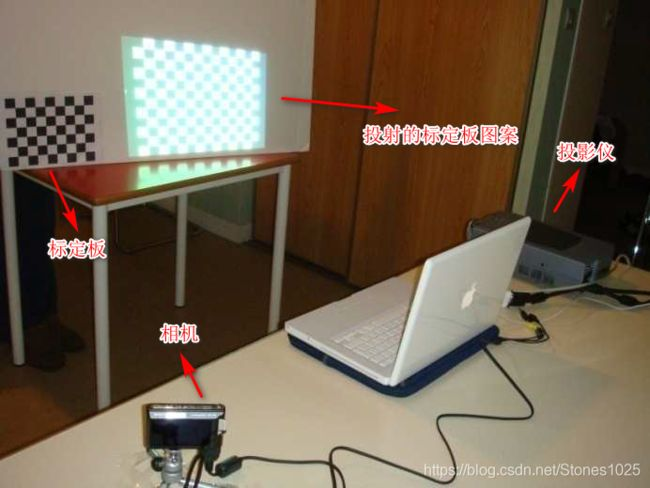2)界面中第一个按钮“Camera Calibration GUI”是用于单相机标定的，而我建议大家是提前标定好的，所以该功能对我们来说没用。点击第二个按钮“Load Camera Calibration”加载自己的相机标定参数(将自己的相机标定参数保存成“Calib_Results.mat”文件放在matlab当前目录中即可)； 4)通常每个按钮点击后都会又对应的提示，根据提示即可获取最终标定结果。 大家好，我主要的研究方向有：1)主流的结构光三维测量方法，包括：线扫，格雷码，相移，散斑等；2)摄像机标定和投影仪标定；3)点云处理；4)图像处理；5)Halcon应用；6)机器视觉相机选型。对以上研究方向感兴趣的朋友可以关注我的微信公众号：另外，关于以上研究方向的任何问题都可以到我的知识星球进行提问，我将给大家满意的回答 ！！！！
展开全文• 本人自己编的 模版也在里面 但是其中主点坐标的y坐标有点不稳定 希望大家帮我看一下 完全能运行
• 自编张正友标定程序，图像角点信息获取需自行解决（不包含角点检测程序）！
• Tsai 经典的两步法标定方法，matlab程序
• 标定相机的参数导出Excel文件和TXT两种格式的程序
• 最近在研究通过Matlab标定程序获得相机内参以及畸变参数之后，如何实现对一张新拍摄的照片进行去畸变。由于Matlab标定程序只能显示标定所用的图片的去畸变图像，并不能直接对由该相机拍摄的一张新图片进行去畸变，...
最近在研究通过Matlab标定程序获得相机内参以及畸变参数之后，如何实现对一张新拍摄的照片进行去畸变。由于Matlab标定程序只能显示标定所用的图片的去畸变图像，并不能直接对由该相机拍摄的一张新图片进行去畸变，所以就想着如何从源程序入手，写一小段代码，脱离标定例程程序，直接调用去畸变源程序。

首先，为了确保调用的源程序可用，大家可以先下载这个工具箱，免费下载地址：http://www.vision.caltech.edu/bouguetj/calib_doc/download/index.html，该工具箱加压后，将其路径添加到Matlab中，可直接调用其中的一些源程序。（该工具箱不是Matlab自带的相机标定，具体使用可以参考：https://www.cnblogs.com/czaoth/p/6708631.html）而本次调用的去畸变源程序主要为工具箱中的rect函数和apply_distortion两个函数。（如果不想下载工具箱，直接赋值本文后面的源程序代码，创建该函数即可）

在已知内参矩阵和畸变参数的情况下去畸变的步骤：

1.首先将像素坐标系转换至相机坐标系

相信大家之前对相机标定都有一定的了解，其中内参矩阵是指摄像机坐标系到图像坐标系再到像素坐标系的转换矩阵。具体为：设摄像机坐标系下的齐次坐标为（x,y,z,1）,像素坐标系的齐次坐标为（u,v,1），则有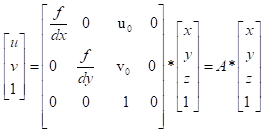则（x,y,z,1)'  =  inv(A)*(u,v,1)'。

2.在相机坐标系下去畸变

由畸变模型：

•xcorrected = x(1+k1r2+k2r4+k3r6)

•ycorrected = y(1+k1r2+k2r4+k3r6) 可得失真图像像素点(x,y)在去畸变之后的位置(xcorrected,ycorrected)，值得注意的是，该过程是对失真图像所有像素点进行操作，并不只是对提取的棋盘格角点进行操作。

其中r2 = x^2+y^2,k1,k2,k3为径向畸变参数，切向畸变在本次应用中不考虑，详细说明可以看这篇博客：https://blog.csdn.net/JennyBi/article/details/85764988

3.将校正后的相机坐标系转回像素坐标系

主要通过下式转换：

u = fx*xcorrected+cx;

v = fy*ycorrected+cy;

4.根据失真图像在校正后的像素坐标位置进行插值处理（赋值）

该程序里插值的方法主要是通过中心像素点左上角(left-up)、右上角(right-up)、左下角(left-down)、右下角的值(right-down)，对中心像素点的亮度值赋值。具体如下：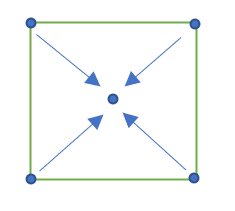a1 = (1 - alpha_y).*(1 - alpha_x);
a2 = (1 - alpha_y).*alpha_x;
a3 = alpha_y .* (1 - alpha_x);
a4 = alpha_y .* alpha_x;

Irec(ind_new) = a1 .* I(ind_lu) + a2 .* I(ind_ru) + a3 .* I(ind_ld) + a4 .* I(ind_rd);

其中alpha_x由步骤三中的u计算得出：alpha_x=u-floor(u);同理，alpha_y=v-floor(v)

至此，去畸变完成。上述过程也就是rect函数的流程，下面给出具体代码参考。

load cameraParams;%加载标定的参数结果
intrinx = cameraParams.IntrinsicMatrix';
I = imread('abc_3.bmp');
I = I(:,:,2);%除此之外，如果对三个通道同时处理，那么出来的就是彩色图

fx = intrinx(1,1);
fy = intrinx(2,2);
fc = [fx fy];
cx = intrinx(1,3);
cy = intrinx(2,3);
cc = [cx cy];
alpha_c = 0;
k21 = cameraParams.RadialDistortion(1);
k22 = cameraParams.RadialDistortion(2);
k23 = cameraParams.RadialDistortion(3);
kc = [k21 k22 k23 0 0];%k1,k2,k3,p1,p2
KK = [fc(1) alpha_c*fc(1) cc(1);0 fc(2) cc(2) ; 0 0 1];
[I2] = rect(double(I),eye(3),fc,cc,kc,alpha_c,KK);%去畸变函数

figure();
image(I2);
colormap(gray(256));
title('Undistorted image');

rect函数

function [Irec] = rect(I,R,f,c,k,alpha,KK_new);

if nargin < 5,
k = [0;0;0;0;0];
if nargin < 4,
c = [0;0];
if nargin < 3,
f = [1;1];
if nargin < 2,
R = eye(3);
if nargin < 1,
error('ERROR: Need an image to rectify');
end;
end;
end;
end;
end;

if nargin < 7,
if nargin < 6,
KK_new = [f(1) 0 c(1);0 f(2) c(2);0 0 1];
else
KK_new = alpha; % the 6th argument is actually KK_new
end;
alpha = 0;
end;

% Note: R is the motion of the points in space
% So: X2 = R*X where X: coord in the old reference frame, X2: coord in the new ref frame.

if ~exist('KK_new'),
KK_new = [f(1) alpha*f(1) c(1);0 f(2) c(2);0 0 1];
end;

[nr,nc] = size(I);%畸变图像尺寸

Irec = 255*ones(nr,nc);%创建去畸变图像尺寸
%% 像素系到相机坐标系的转换
[mx,my] = meshgrid(1:nc, 1:nr);
px = reshape(mx',nc*nr,1);%将mx’按列顺序，将其变为nc*nr行，1列的矩阵
py = reshape(my',nc*nr,1);

rays = inv(KK_new)*[(px - 1)';(py - 1)';ones(1,length(px))];

% Rotation: (or affine transformation):

rays2 = R'*rays;

x = [rays2(1,:)./rays2(3,:);rays2(2,:)./rays2(3,:)];
%% 在相机坐标系下去畸变（主要是找失真像素点在未失真情况下上的坐标）

% Add distortion:
xd = apply_distortion(x,k);

%% 将相机坐标系转回像素坐标系
% Reconvert in pixels:

px2 = f(1)*(xd(1,:)+alpha*xd(2,:))+c(1);
py2 = f(2)*xd(2,:)+c(2);

%% 对像素值进行插值处理
% Interpolate between the closest pixels:

px_0 = floor(px2);

py_0 = floor(py2);
py_1 = py_0 + 1;

good_points = find((px_0 >= 0) & (px_0 <= (nc-2)) & (py_0 >= 0) & (py_0 <= (nr-2)));

px2 = px2(good_points);
py2 = py2(good_points);
px_0 = px_0(good_points);
py_0 = py_0(good_points);

alpha_x = px2 - px_0;
alpha_y = py2 - py_0;

a1 = (1 - alpha_y).*(1 - alpha_x);
a2 = (1 - alpha_y).*alpha_x;
a3 = alpha_y .* (1 - alpha_x);
a4 = alpha_y .* alpha_x;

ind_lu = px_0 * nr + py_0 + 1;
ind_ru = (px_0 + 1) * nr + py_0 + 1;
ind_ld = px_0 * nr + (py_0 + 1) + 1;
ind_rd = (px_0 + 1) * nr + (py_0 + 1) + 1;

ind_new = (px(good_points)-1)*nr + py(good_points);

Irec(ind_new) = a1 .* I(ind_lu) + a2 .* I(ind_ru) + a3 .* I(ind_ld) + a4 .* I(ind_rd);

return;

% Convert in indices:

fact = 3;

[XX,YY]= meshgrid(1:nc,1:nr);
[XXi,YYi]= meshgrid(1:1/fact:nc,1:1/fact:nr);

%tic;
Iinterp = interp2(XX,YY,I,XXi,YYi);
%toc

[nri,nci] = size(Iinterp);

ind_col = round(fact*(f(1)*xd(1,:)+c(1)))+1;
ind_row = round(fact*(f(2)*xd(2,:)+c(2)))+1;

good_points = find((ind_col >=1)&(ind_col<=nci)&(ind_row >=1)& (ind_row <=nri));

apply_distort函数

function [xd,dxddk] = apply_distortion(x,k)

% Complete the distortion vector if you are using the simple distortion model:
length_k = length(k);
if length_k <5 ,
k = [k ; zeros(5-length_k,1)];
end;

[m,n] = size(x);

% Add distortion:

r2 = x(1,:).^2 + x(2,:).^2;

r4 = r2.^2;

r6 = r2.^3;

% Radial distortion:

cdist = 1 + k(1) * r2 + k(2) * r4 + k(3) * r6;
% cdist = 1 + k(1) * r2 + k(2) * r4 + k(5) * r6;%原版

if nargout > 1,
dcdistdk = [ r2' r4' zeros(n,2) r6'];
end;

xd1 = x .* (ones(2,1)*cdist);

coeff = (reshape([cdist;cdist],2*n,1)*ones(1,3));

if nargout > 1,
dxd1dk = zeros(2*n,5);
dxd1dk(1:2:end,:) = (x(1,:)'*ones(1,5)) .* dcdistdk;
dxd1dk(2:2:end,:) = (x(2,:)'*ones(1,5)) .* dcdistdk;
end;

% tangential distortion:

a1 = 2.*x(1,:).*x(2,:);
a2 = r2 + 2*x(1,:).^2;
a3 = r2 + 2*x(2,:).^2;

% delta_x = [k(3)*a1 + k(4)*a2 ;
%    k(3) * a3 + k(4)*a1];%原版
delta_x = [k(4)*a1 + k(5)*a2 ;
k(4) * a3 + k(5)*a1];

aa = (2*k(3)*x(2,:)+6*k(4)*x(1,:))'*ones(1,3);
bb = (2*k(3)*x(1,:)+2*k(4)*x(2,:))'*ones(1,3);
cc = (6*k(3)*x(2,:)+2*k(4)*x(1,:))'*ones(1,3);

if nargout > 1,
ddelta_xdk = zeros(2*n,5);
ddelta_xdk(1:2:end,3) = a1';
ddelta_xdk(1:2:end,4) = a2';
ddelta_xdk(2:2:end,3) = a3';
ddelta_xdk(2:2:end,4) = a1';
end;

xd = xd1 + delta_x;

if nargout > 1,
dxddk = dxd1dk + ddelta_xdk ;
if length_k < 5,
dxddk = dxddk(:,1:length_k);
end;
end;

return;

% Test of the Jacobians:

n = 10;

lk = 1;

x = 10*randn(2,n);
k = 0.5*randn(lk,1);

[xd,dxddk] = apply_distortion(x,k);

% Test on k: OK!!

dk = 0.001 * norm(k)*randn(lk,1);
k2 = k + dk;

[x2] = apply_distortion(x,k2);

x_pred = xd + reshape(dxddk * dk,2,n);

norm(x2-xd)/norm(x2 - x_pred)

实验结果

失真图像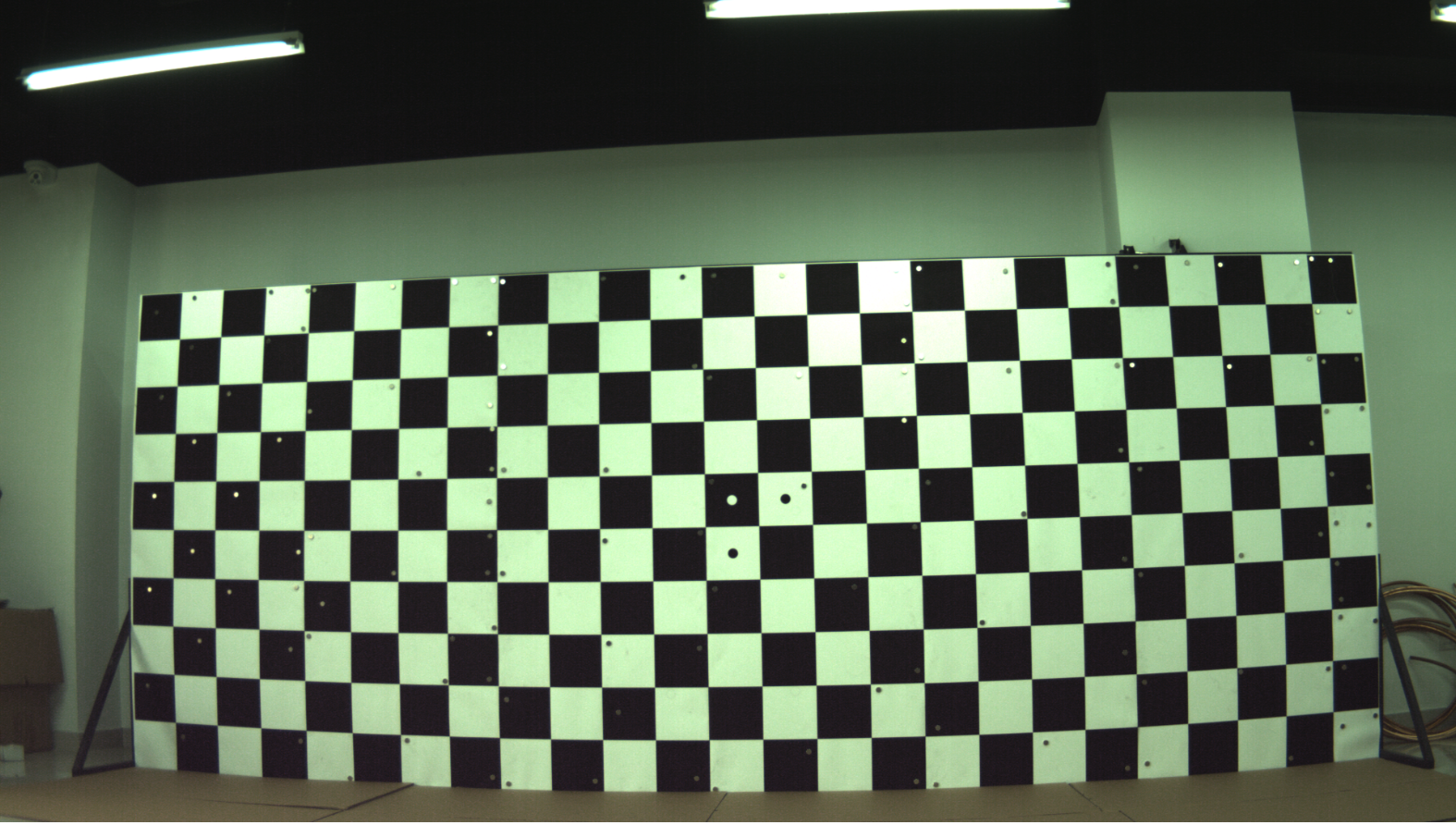去畸变之后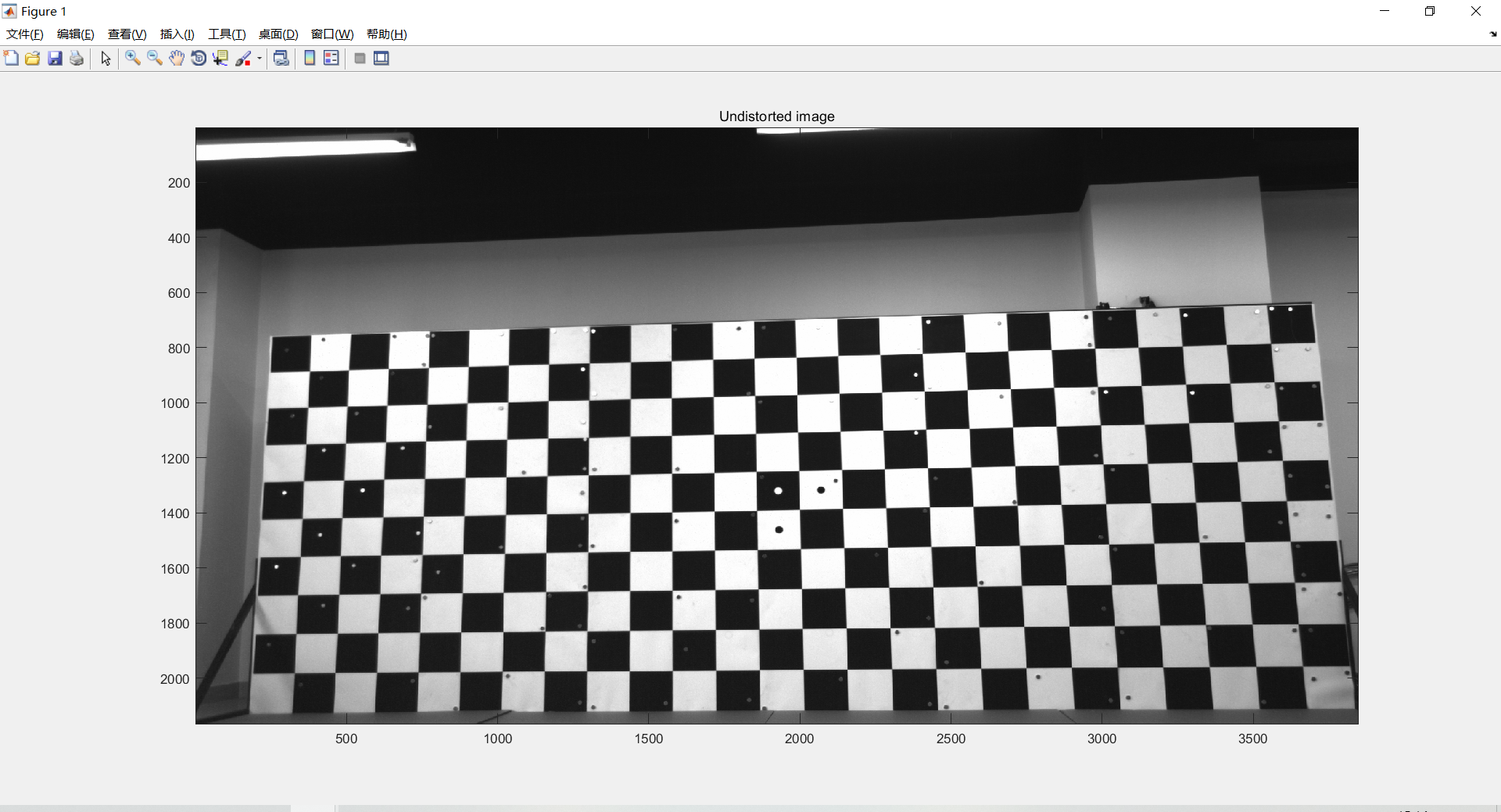展开全文计算机视觉
• ## 相机标定Matlab程序

热门讨论 2010-03-19 10:18:54
Jean-Yves Bouguet写的相机标定程序，超级人性化，超级好用，按照提示操作即可，压缩文件中包括示例图像。
• 在我们进行机械臂抓取实验时候，总是少不了相机的标定环节。我认为相机标定可以分为两个部分。一个部分是相机外参的标定，也就是获得相机坐标系与世界坐标系的关系，在我们的系统中，世界坐标系是可以自定义的。其中...


在我们进行机械臂抓取实验时候，总是少不了相机的标定环节。我认为相机标定可以分为两个部分。一个部分是相机外参的标定，也就是获得相机坐标系与世界坐标系的关系，在我们的系统中，世界坐标系是可以自定义的。其中眼在手上的标定，不变的是相机与机械臂末端的坐标转换。通过手眼标定获得这两者的转换，结合机械臂TF树，便可以相机坐标与机械臂基座标的变换，进而获得相机坐标与世界坐标系的变换。而眼在手外的相机标定可以直接获得相机坐标与机械臂基座标的变换，进而获得相机坐标与世界坐标的关系，完成相机外参标定过程。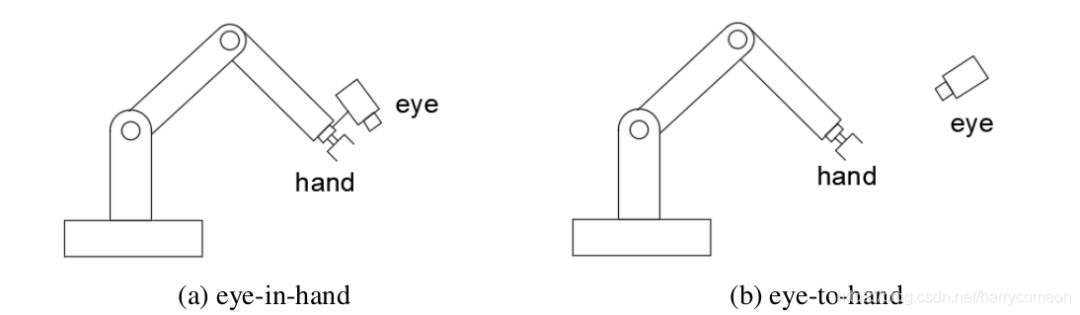另一部分是相机内参的标定，之前有在matlab环境下进行相机的内参标定，那么为什么要进行内参标定呢？其实这里的相机标定主要为了解决空间中物体表面的三维几何点与其相对应的图像点的转换关系。下面我将介绍一下相机内参标定的原理，通过介绍可以理解为什么进行内参标定。相机成像模型相机的成像过程是将三维空间中的物体投影到二维图像空间的，即透视投影变换，也被称之为小孔成像。相机的成像模型涉及像素坐标系、图像坐标系、相机坐标系以及世界坐标系之间的坐标转换关系，各坐标系如图所示。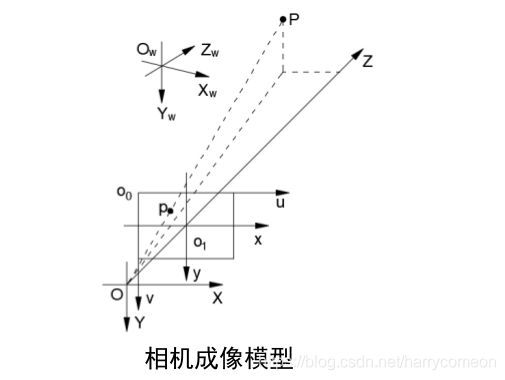相机模型坐标定义像素坐标系u，v轴组成，位于像素平面上,其原点位于图像左上角，单位为像素。图像坐标系x，y轴组成，位于成像平面上,原点在在成像平面的中心点附近，单位为物理单位(如 mm)。相机坐标系 X，Y，Z轴组成，原点为光心，单位为物理单位。世界坐标系 Xw ，Yw， Zw 组成，是实际三维空间中的坐标系，根据使用需要进行定义，是用于表示系统中绝对位置的坐标系，单位为物理单位(如 mm)。像素与图像坐标转换像素坐标系和图像坐标系之间的转换关系可以通过单个像素的物理尺寸来关联: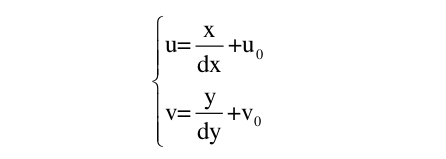其中, dx和dy表示沿x和y轴方向上单个像素点的实际物理尺寸,与感光芯片有关, (u0,v0)为原点o1在像素坐标系下的坐标值。将上式用齐次坐标系表示：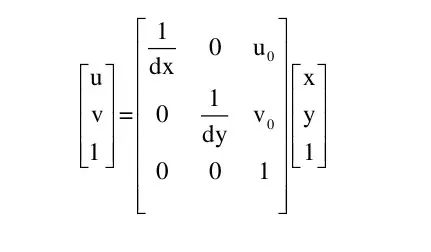相机与世界坐标转换通过相机的外参标定，世界坐标系下的坐标可以通过旋转矩阵R和平移向量t转换到相机坐标系下：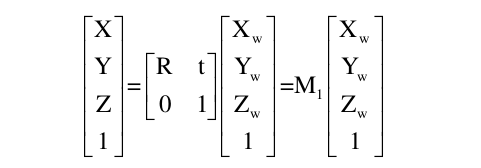式中矩阵R为 3×3的正交单位阵,向量t表示3×1的三维平移量。相机与图像坐标转换对于三维空间中的一点P,其对应在图像平面上的成像点为P与光心O的连线与图像平面的交点 p，O与o1之间的距离为焦距f 。根据三角形相似定理可以推出相机坐标系与图像坐标的关系满足以下关系：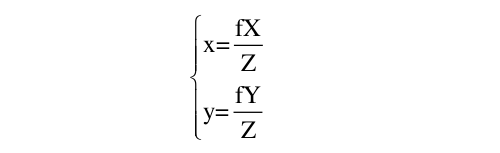将上式用齐次坐标系表示：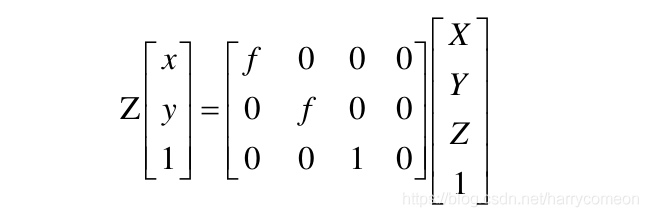像素与世界坐标转换将式5和式5带入式2中可以计算出世界坐标系下的一点 P(Xw , Yw , Zw) 在像素坐标系下的成像点 p(u,v) :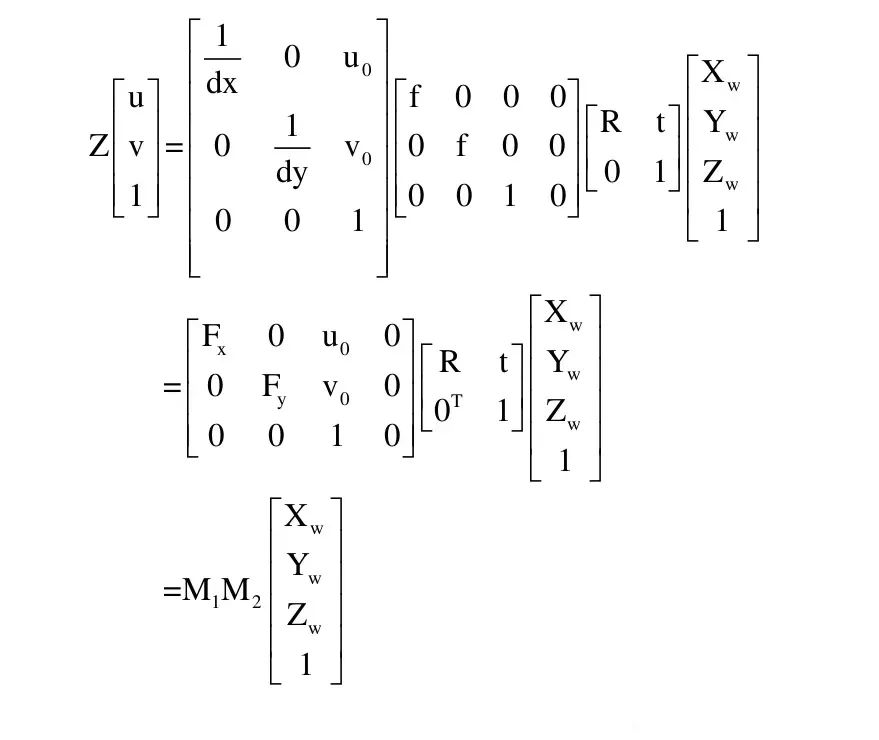畸变其中, Fx 和 Fy 被称为等效焦距, M1为相机内参只与相机内部结构有关, M2为相机外参表示相机坐标系与世界坐标系之间的转换。上述的相机模型为理想条件下的相机成像模型,而实际的成像过程往往存在偏差,即畸变。畸变又可以进一步划分为径向畸变以及切向畸变,径向畸变来源于相机透镜在制造过程中的误差,而切向畸变则来源于整个相机的组装误差。产生两者畸变的原理图：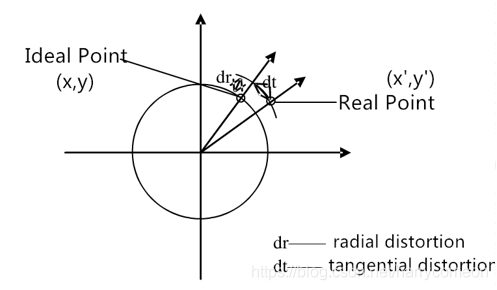径向畸变矫正的表达式为: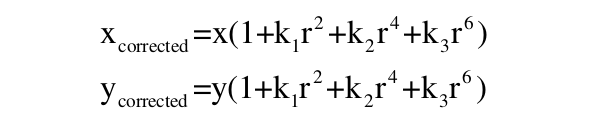其中,r 为图像坐标 (x, y) 到其原点的欧式距离, k1 、 k2 和 k3 为畸变系数。径向畸变包括：枕形畸变、桶形畸变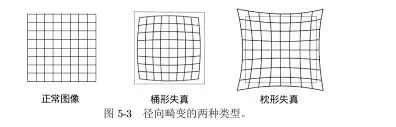切向畸变矫正的表达式为：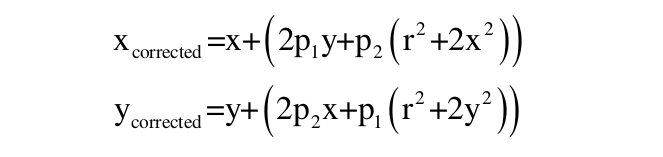其中, p1和p2为畸变系数。k1 、k2 、k3 、p1 、p2 和 M1共同构成了相机的内参。切向畸变图示：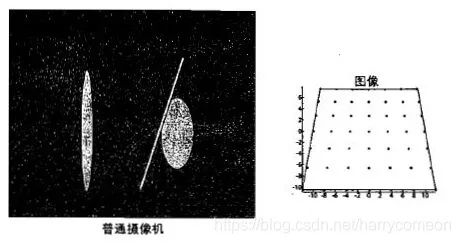(本文经授权转载自CSDN，作者我是。，未经授权禁止二次转载与摘编。)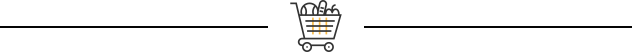《ROS常用SLAM功能包使用指南 · 古月》课程将带你走进ROS的SLAM世界，了解SLAM的基本原理，熟悉ROS中常用SLAM功能包的配置及使用方法。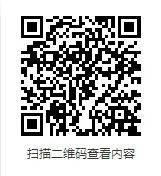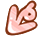展开全文•  用VS2012+OpenCV2.4.6写的双目标定的程序（其实就是跑的Samples），实验结果一直很不理想，查看很多Blog，说可以先用MATLAB标定工具箱（http://download.csdn.net/detail/hyacinthkiss/8180789）获得extrinsics和...

MATLAB标定工具箱的使用

用VS2012+OpenCV2.4.6写的双目标定的程序（其实就是跑的Samples），实验结果一直很不理想，查看很多Blog，说可以先用MATLAB标定工具箱（http://download.csdn.net/detail/hyacinthkiss/8180789）获得extrinsics和intrinsics矩阵，然后作为输入参数再读到OpenCV的stereoRectify函数，进而就可以Match了。这里，分享一下我做MATLAB标定的过程：
p.s.详细的用法可以见：http://www.vision.caltech.edu/bouguetj/calib_doc/htmls/example.html

一、环境配置

1.解压MATLAB标定工具箱至某个文件夹，默认为：TOOLBOX_calib，将之存放在MATLAB\R2010b\toolbox下（个人喜欢，也可以放其他位置）。

2.运行Matlab并添加文件夹TOOLBOX_calib的位置到matlab路径path中，具体操作为：File->Set Path->Add Folder To Path，然后找到刚刚存放的文件夹TOOLBOX_calib，save一下就OK了。

二、文件配置

将所有要标定的棋盘图放到文件夹TOOLBOX_calib里.m文件所在的目录下（个人喜欢将左右相机的图片命名为left01.jpg和right01.jpg等的形式，看着舒服，也和后面要讲的标定结果保存对应起来），OK，万事俱备，准备开动了。

三、单目标定

1.在Matlab命令行窗口中输入calib_gui，出现以下窗口。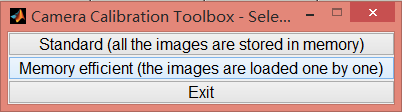2.选择Standard之后便出现以下窗口。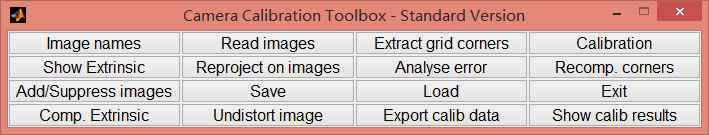3.点击Image names，命令行窗口会提示你输入图片的basename以及图片的格式（比如你图片文件名是right01, right02, …, right12，basename就是right，图片格式如：jpg），然后Matlab会自动帮你读入这些图片，如下图所示，可以看到，读入了12幅右摄像头的棋盘图。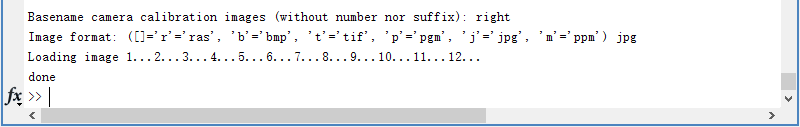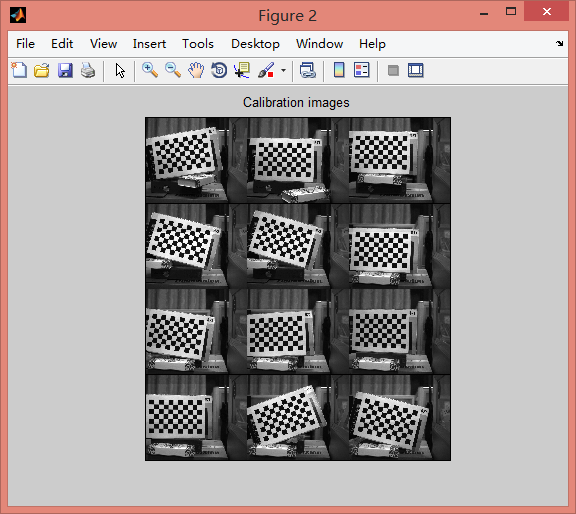4.然后再回到主控制界面，点击Extract grid corners，提取每幅图的角点。点击完后，命令行会出现如下提示，主要是让你输入棋盘角点搜索窗口的大小。如果窗口定大一点的话提取角点会比较方便点，即便点得偏离了也能找到，但也要注意不能大过一个方格的大小。这些选项，都只要敲回车选用默认设置就可以了。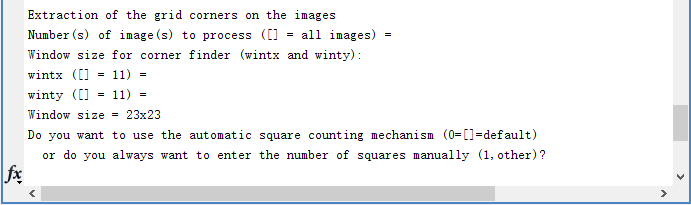5.继上步Enter敲完后，跳出第一幅棋盘格图，按顺时针或是逆时针方向分别点选棋盘格的最外面的四个内格点，程序会自动检测出其余的角点。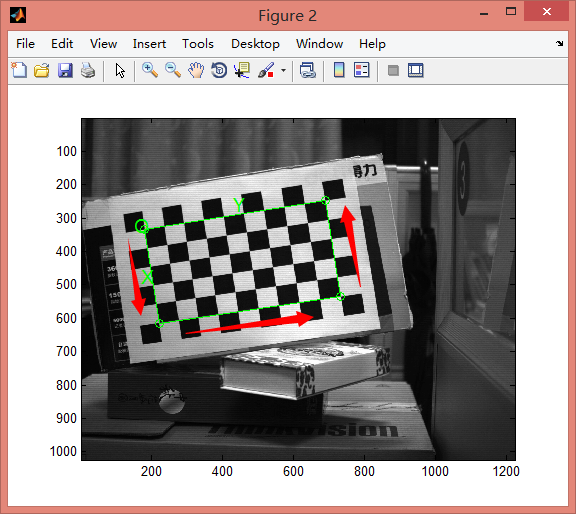6.在选完四个角点后，程序会在命令行窗口提示你输入标定板棋盘的size，输入你棋盘格的实际大小就行，比如我的棋盘格是24mm，就输入24。这步事实上相当关键，它定义了空间的尺度，如果要对物体进行测量的话，这步是必须的。Ender后同时得到角点检测结果图。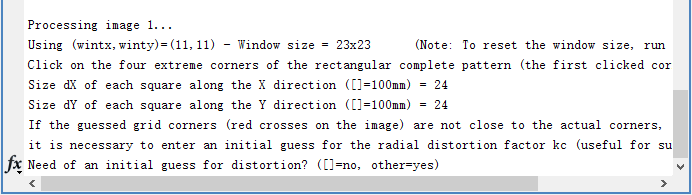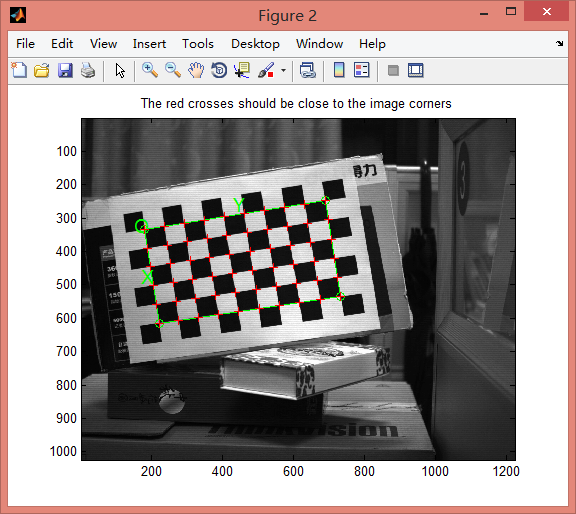7.继续命令行窗口按Ender选择默认项，跳出新的标定板图像，同样的方法，如此反复，检测角点完12幅左视图。

8.检测完所有的图像后，点击Calibration，开始摄像头标定。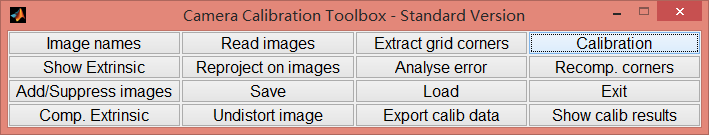9.标定完成后，可以进行各种结果的可视化观察。由Show Extrinsic得的结果图可见相机与标定板的距离约为1000mm左右，且其中各种颜色标定板对应Analyse Error的结果图的各种误差结果，其中，红色的（对应第2幅标定板图像）的误差较大，部分角点提取有问题（这是当时选角点时我故意选得偏离了一些，验证一下）。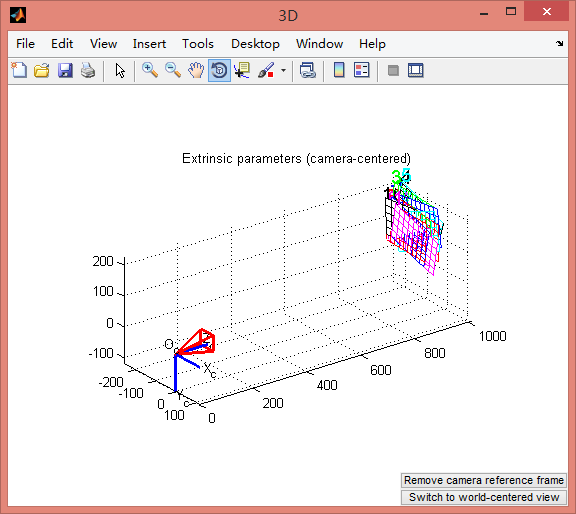图1.Show Extrinsic的结果图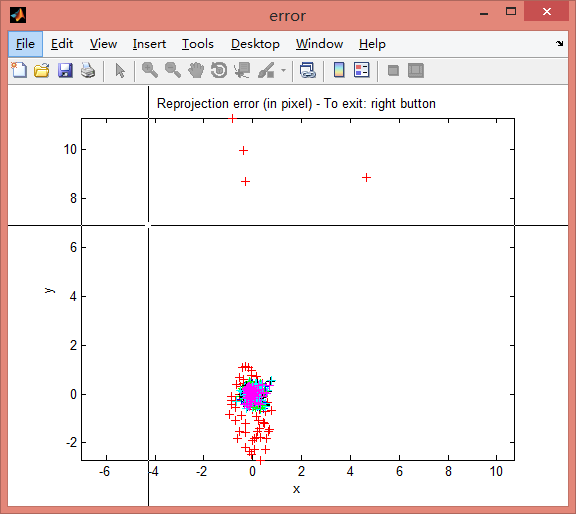图2.Analyse Error的结果图

10.验证标定结果无误之后，就点击面板上的Save按钮，程序会把标定结果放在一个叫Calib_Result.mat中，默认存储路径为：文件夹TOOLBOX_calib里.m文件所在的目录下。为了方便后续立体标定，把这个文件名改为Calib_Result_right.mat。

11.左摄像头标定的方法与右摄像头相同，生成的Calib_Result.mat之后，将其改名为Calib_Result_left.mat就可以了。

四、双目标定

1.左右摄像头都标定完成之后，就可以开始立体标定了。在Matlab命令行中键入stereo_gui启动立体标定面板，如下图所示。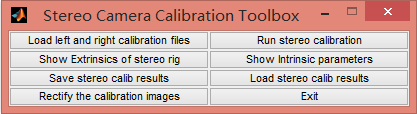2.点击Load left and right calibration files，在命令行窗口中会提示你分别填写左右自标定的结果，如果你按上面的文件名保存方式的话，直接Ender选择默认的文件名(Calib_Result_left.mat和Calib_Result_right.mat)，或是填写你自己保存的文件名。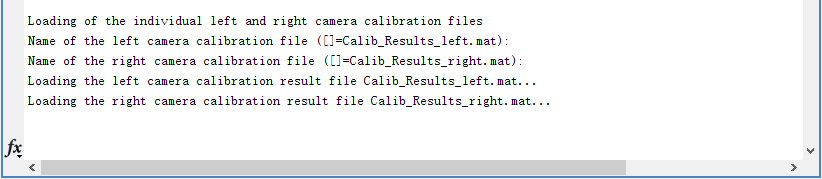3.Load成功后，就可以开始Run stereo calibration了，run之后的结果如下图所示，左右摄像头的参数都做了修正，并且也求出了两个摄像头之间的旋转和平移关系向量(om和T)。从结果可以看出左右相机的Focal Length相差还是蛮大的，不知这对后续的视差图等计算影响如何（怎么调整两个摄像机的焦距相同也是个问题）。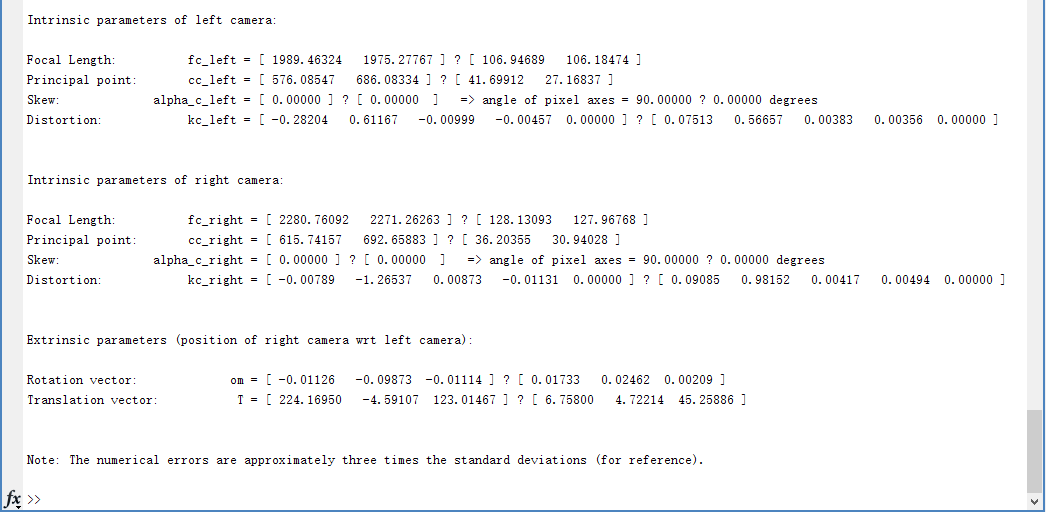4.run成功后，就可以看标定的结果了。同样可以直观的看出相机的相对位置相差很大。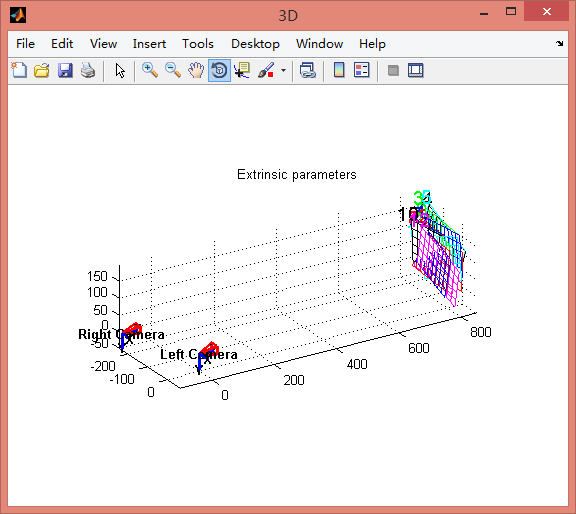图3.Show Extrinsic of stereo rig的结果图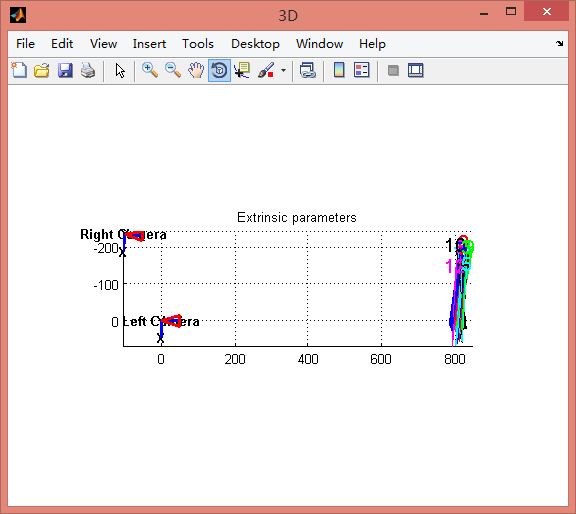图4.图3的俯视图

5.还可以通过Rectify the calibration images按钮，得到纠正后的所有棋盘格图片，可以直接读入OpenCV中进行Match了。当然，你需要对其他物体或是想实时得到视差图，还得将互标定结果写到OpenCV函数调用时支持的格式。

五、问题探讨

1.相机的焦距是否应该保持一致？

答：答案是应该肯定的。因为在后续的视差图转换为三维图时的Q矩阵只有一个f值。所以必须要求至少焦距相近。而且立体成像的三角测量（Learning OpenCV书中提到）的前提假设就是fl=fr。不知道理解的对不对。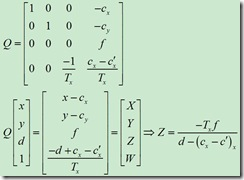2.怎么调整两个摄像机的焦距相同？

答：a.离两个相机相同远处放置标定板（可以将两个相机放置在一起以打到这个效果），分别调节两个相机的焦距，使得两个画面的清晰度相似。（这是我咨询大恒图像的技术支持的结果）

b.我觉得这样肯定还是不行，可以再微调，就是标定一次得一个焦距，再微调一下，再测，这样就会很麻烦。

不知有没有更好的方法。看了很多Blog，没看到涉及这块的，不知道大家都是怎么解决的。

展开全文• 为什么需要相机标定 标定是为了能够从空间点的像素坐标映 射到世界坐标，这是3D立体视觉必须经过的过程。 相机标定的四个坐标系 1.1.1. 相机坐标与世界坐标系转换： 1.1.2. 相机坐标系与像平面坐标系...计算机视觉
• 使用最小二乘拟合对陀螺仪的零偏、标度因数和三轴不重合误差进行标定，很基础的算法。使用最小二乘拟合对陀螺仪的零偏、标度因数和三轴不重合误差进行标定，很基础的算法。
• 单目相机标定matlab代码，ubuntu操作系统下实验，标定效果理想
• 陀螺仪静态标定matlab程序，六位置法，希望对大家有帮助
• 后来请教学长后改用matlab标定，解决了映射畸变问题 具体的matlab标定方法可以看下面三个大佬的博文，亲测可以 http://blog.csdn.net/dreamharding/article/details/53700166  可以用来把.mat文件转成.xml ...
• 在 http://www.vision.caltech.edu/bouguetj/calib_doc/ 提供了一个很好的matlab标定工具箱，但在使用中我发现由于原工具箱的目录设置问题，常常在第一步卡住程序无法运行，因此我对程序进行了更改，更改后的标定...工具
• 旷视SLAM组主要负责多传感器建图、定位、标定等工作，在开发过程中总结了一些理论和实践方面的经验教训，在此分享出来，希望能够与大家一起探讨，为机器人行业贡献自己的绵薄之力。近期我们会首先推出机器人多传感器...
• 最近做科研用到手眼系统标定，在网上搜索方法无果，于是自己亲自编写手眼标定程序，经验证，具有较高精度。...

# matlab标定程序matlab 订阅